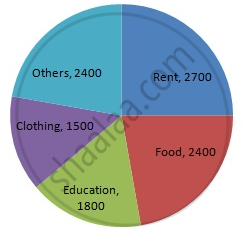Share

# The Following Data Shows the Expenditure of a Person on Different Items During a Month. Represent the Data by a Pie-chart. - Mathematics

Course
ConceptCircle Graph Or Pie Chart - Drawing Pie Charts

#### Question

The following data shows the expenditure of a person on different items during a month. Represent the data by a pie-chart.

 Items of expenditure Rent Education Food Clothing Others Amount (in Rs) 2700 1800 2400 1500 2400

#### Solution

We know:
Central angle of a component = (component value/sum of component values x 360)
Here, total amount = Rs 10800
Thus, the central angle for each component can be calculated as follows:

 Item Amount (in Rs) Sector angle Rent 2700 2700/10800 x 360 = 90 Education 1800 1800/10800 x 360 = 60 Food 2400 2400/10800 x 360 = 80 Clothing 1500 1500/10800 x 360 = 50 Others 2400 2400/10800 x 360 = 80

Total : 10800 (in Rs)

Now, the pie chat representing the given data can be constructed by following the steps below:
Step 1 : Draw circle of an appropriate radius.
Step 2 : Draw a vertical radius of the circle drawn in step 1.
Step 3 : Choose the largest central angle. Here, the largest central angle is 90o. Draw a sector with the central angle 90o in such a way that one radius coincides with the radius drawn in step 2 and another radius is in its counter clockwise direction.
Step 4 : Construct other sectors representing other items in the clockwise direction in descending order of magnitudes of their central angles.
​Step 5 : Shade the sectors with different colours and label them, as shown as in the figure below.​​Is there an error in this question or solution?

#### APPEARS IN

RD Sharma Solution for Mathematics for Class 8 by R D Sharma (2019-2020 Session) (2017 to Current)
Chapter 25: Data Handling-III (Pictorial Representation of Data as Pie Charts or Circle Graphs)
Ex. 25.1 | Q: 4 | Page no. 12

#### Video TutorialsVIEW ALL 

Solution The Following Data Shows the Expenditure of a Person on Different Items During a Month. Represent the Data by a Pie-chart. Concept: Circle Graph Or Pie Chart - Drawing Pie Charts.
S